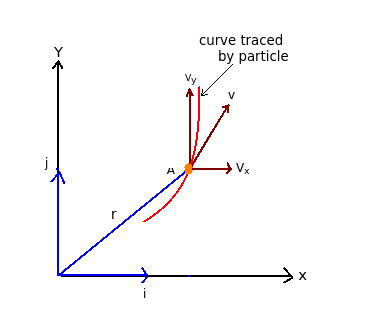# Curvilinear motion - Rectangular components of velocities

Q.  What are the rectangular components of velocities in curvilinear motion?
- Published on 18 Sep 15

a. dx/dt and dy/dt
b. dr/dt and d2r/dt
c. d2x/dt2 and d2y/dt2
d. none of the above

#### Discussion

• Sravanthi   -Posted on 15 Dec 15- In the rectangular co-ordinate system shown above, particle A travels the curved path. The tangential component of velocity is equal to velocity itself.

- Vx and Vy are the velocity co-ordinates of particle A.

V = (Vx)i + (Vy) j

(Vx)i is the horizontal component and (Vy) is the vertical component.

(Vx) = dx/dt and (Vy) = dy/dt

## ➨ Post your comment / Share knowledgeEnter the code shown above:

(Note: If you cannot read the numbers in the above image, reload the page to generate a new one.)